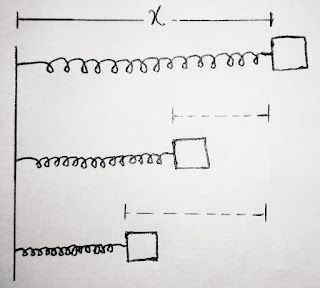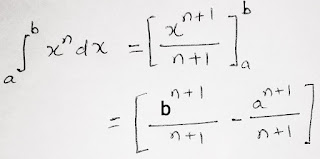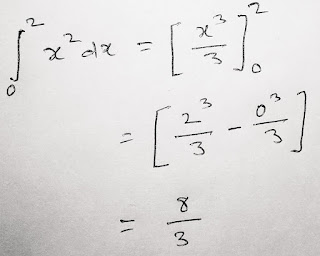# Integration for Physics ( Application ) by A.K.Sinha

Integration for Physics

( Application ) :

(Click here for : Electric Potential due to a point charge by Integration method)

A simple , short & simplified concept of integration :

Integration is a mathematical operation which is used for continuous summation as the normal addition operation is used for discrete summation.

We can proceed with it as the sum of products of two quantities such that the first quantity is independent while the other quantity is dependent on the first quantity.

Now let's  understand the concept under which we use the operation of integration in Physics. we consider a spring block system and we are trying to compress the spring by applying a force on the block.As we are applying the force, the spring is compressed such that the force we are applying is continuously increasing as to further compress the spring. In this process the displacement is the independent quantity while the force is the dependent quantity which is continuously varying with displacement. And in this case  if we try to find out the work done then we find first the small amount of work done by each value of varying force at every instant during total displacement and then add all small amount of continuous work done.

We can also understand it as if we try to find area of given graph.In this case we use Integration method to find area under the curve as value of y for the curve is varying with displacement. The process under integration is as follows:

We first consider a strip of very small width dx as shown in fig. As the strip is very thin the shape of strip is almost a rectangle,so we can calculate the area of that strip given by

dA1 = y1dx………….(1)

Similarly, the next strip is considered continuously just after first one, we can write it further

dA2 = y2 dx…………(2)

And so on..

In short, we can say that we have to move a strip with varying value of y according to curve between 0 to x and then add all small continuous areas to find the total area as

A = dA1 + dA2 + dA3 + …..

and since the considered areas are continuous, so we write the above summation in the form of integration as

A = 0x dA = 0x y dx

Here the symbol is for integration and we read the above equation as the total area under the curve is integration of dA, which is equal to integration of y with respect to dx between 0 to x ( 0 and x are the lower and upper limits for integration respectively, i.e y is varying with respect to displacement, as from displacement = 0 to displacement = x) .

IMPORTANT FORMULAS FOR INTEGRATION :

•  ∫ xn dx = (xn+1) /(n+1) + C

( here C is a constant of integration that we don't use in definite integration where we consider two limits for integration )

Example : ( how to put the limit in definite Integration )Here we put x = b once and then x = a (where, a and b are the upper and lower limit for the integration respectively) and then we find the difference between two as shown above.

Let's understand with an example:Other important formulas ( useful in physics) :

•  ∫ 1/x dx = log | x | + C

•  ∫ dx = x + C

•  ∫ a dx = a ∫ dx = ax +C ( where 'a' is a constant value )

•  ∫ cosx dx = sinx + C

•  ∫ sinx dx  = - cosx + C

( NOTE : All above formulas are for indefinite integration as we used here the constant of integration. And in definite integral we don't consider any constant as the limits are already mentioned in it.)